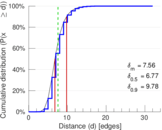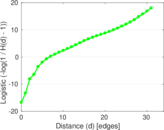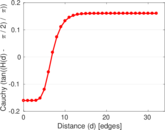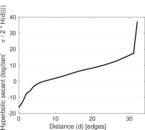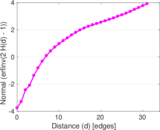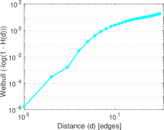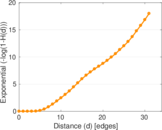# UK domain (2002)

This is the hyperlink network of the .uk domain for the United Kingdom.

 Code `UD` Internal name `dimacs10-uk-2002` Name UK domain (2002) Data source https://www.cc.gatech.edu/dimacs10/archive/clustering.shtml AvailabilityDataset is available for download Consistency checkDataset passed all tests Category Hyperlink network Dataset timestamp 2002 Node meaning Page Edge meaning Hyperlink Network format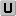Unipartite, undirected Edge typeUnweighted, no multiple edges LoopsDoes not contain loops Snapshot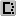Is a snapshot and likely to not contain all data Orientation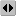Is not directed, but the underlying data is Multiplicity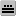Does not have multiple edges, but the underlying data has

## Statistics

 Size n = 18,483,186 Volume m = 261,787,258 Loop count l = 0 Wedge count s = 201,459,939,137 Claw count z = 1,982,637,974,174,774 Cross count x = 7.010 62 × 1019 Triangle count t = 4,451,687,605 Maximum degree dmax = 194,955 Average degree d = 28.327 1 Size of LCC N = 18,459,128 Diameter δ = 45 50-Percentile effective diameter δ0.5 = 6.765 60 90-Percentile effective diameter δ0.9 = 9.776 62 Median distance δM = 7 Mean distance δm = 7.564 49 Balanced inequality ratio P = 0.234 540 Clustering coefficient c = 0.066 291 4 Spectral norm α = 943.000 Non-bipartivity bA = 0.141 624

## Plots

### Cumulative degree distribution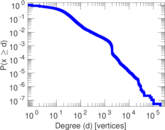### Hop distribution